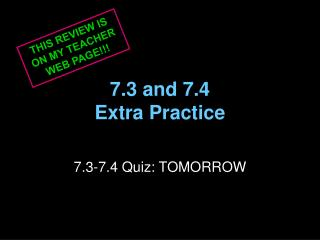DownloadDownload Presentation7.3 and 7.4 Extra Practice

# 7.3 and 7.4 Extra Practice

Download Presentation## 7.3 and 7.4 Extra Practice

- - - - - - - - - - - - - - - - - - - - - - - - - - - E N D - - - - - - - - - - - - - - - - - - - - - - - - - - -
##### Presentation Transcript

1. THIS REVIEW IS ON MY TEACHER WEB PAGE!!! 7.3 and 7.4Extra Practice 7.3-7.4 Quiz: TOMORROW

2. Section 7.3 • Find the mean, median, and mode of the data set • 12, 15, 17, 11, 8, 14, 7, 14 x = 12.25, Median = 13, Mode = 14 • 0.3, 8.9, 7.6, 3.7, 1.5, 4.2, 7.6, 8.1 x = 5.2375, Median = 5.9, Mode = 7.6

3. Section 7.3 • Find the range and standard deviation of the data set. • 1.25, 3.69, 5.67, 4.89, 0.12, 4.35, 2.78 Range = 5.55, Standard Deviation = about 1.8 • 515, 720, 635, 895, 585, 690, 770, 840 Range = 380, Standard Deviation = about 119.6

4. Standard Deviation

5. Section 7.4 • A normal distribution has a mean x and standard deviation σ. Find the indicated probability for a randomly selected x-value from the distribution. • P(x < x + 2σ) 97.5% • P(x > x – σ) 84% • P( x - 3σ< x < x +σ) 83.85%

6. Section 7.4 • Give the percent of the area under the normal curve represented by the shaded region. 2.5% 50%

7. Section 7.4 • A normal distribution has a mean of 63.7 and a standard deviation of 2.9. Find the probability that a randomly selected x-value from the distribution is in the given interval. • Between 57.9 and 66.6 81.5% • At most 69.5 97.5%

8. Section 7.4 • A normal distribution has a mean of 496 and a standard deviation of 109. Use the standard normal table on p. 296 to find the indicated probability for a randomly selected x-value from the distribution. • P(x < 630) .8849 88.49% • P(x < 270) .0179 1.79%